B4R LibraryrLCDBitmap

It is a wrap for this Github project. Quite old but nevertheless very nifty.
See the detail usage of the library here.

Note the following:
".....It works by creating a memory array for the 20x16 pixel bitmap that the functions work within. It then converts this bitmap to the 8 custom characters available with the HD44780 and displaying them as 2 rows of 4 characters at the location specified. As each character is 5x8 pixels and arranged in a 4x2 character array, the total addressable resolution is 20x16. As there's an 8 custom character limit with the HD44780, 20x16 is the maximum resolution that can be achieved (5*4=20 X 8*2=16)....."

Attached the B4R lib files. Copy the folder with its contents to the root of your B4R additional libs folder. Copy rLCDBitmap.xml to the root of your B4R additional libs folder.

Popup help available in the library.

Sample B4R project attached
Wiring diagram: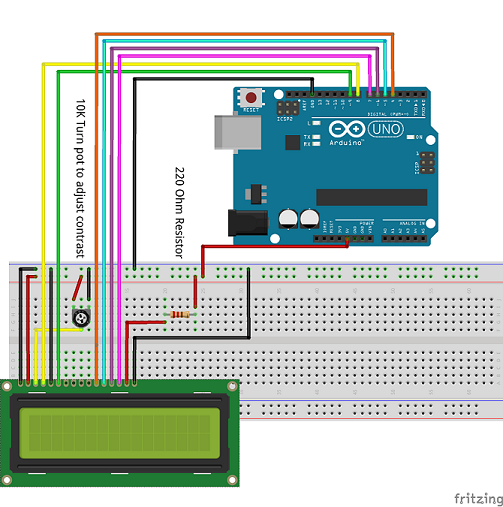Snapshot: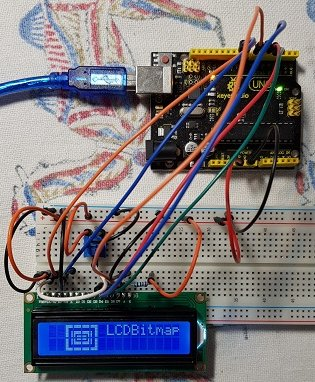Sample code:
B4X:
#Region Project Attributes
#AutoFlushLogs: True
#CheckArrayBounds: True
#StackBufferSize: 300
#End Region

Sub Process_Globals
'These global variables will be declared once when the application starts.
'Public variables can be accessed from all modules.
Public Serial1 As Serial
Dim lcd As LiquidCrystal                                 'rLiquidCrystal must be enabled in the B4R IDE
Dim bitmap As LcdBitmap                                  'rLCDBitmap must be enabled in the B4R IDE
Dim t As Timer
Dim cnt As Byte = 0

End Sub

Private Sub AppStart
Serial1.Initialize(115200)
Log("AppStart")
t.Initialize("t_tick", 2000)

lcd.Initialize(8,255,9, Array As Byte(4,5,6,7))          'initialize the LCD (rLiquidCrystal) library
lcd.Begin(16,2)
bitmap.Initialize(lcd, 0, 0)
bitmap.begin

lcd.SetCursor(7, 0)
lcd.Write("LCDBitmap")

bitmap.rectFill(0, 0, 19, 15, bitmap.COLOR_ON, bitmap.UPDATE_OFF)
bitmap.rectFill(2, 2, 17, 13, bitmap.COLOR_OFF, bitmap.UPDATE_OFF)
bitmap.rectFill(4, 4, 15, 11, bitmap.COLOR_ON, bitmap.UPDATE_OFF)
bitmap.rect(6, 6, 13, 9, bitmap.COLOR_OFF, bitmap.UPDATE_ON)

t.Enabled = True

End Sub

Sub t_tick
cnt = cnt + 1
If cnt = 1 Then
bitmap.move(1, 0)
else if cnt = 2 Then
bitmap.move(2,0)
else if cnt = 3 Then
bitmap.move(3,0)
bitmap.clear_text
Else
bitmap.move(0,0)
lcd.SetCursor(7, 0)
lcd.Write("LCDBitmap")
cnt = 0
End If
End Sub

Will post another couple of samples with what the library can do.

Attachments

• b4rLCDBitmap.zip
1.2 KB · Views: 194
• rLCDBitmap.zip
97.3 KB · Views: 180
Last edited:

Johan Schoeman

Expert
b4rLCDBitmapGraph sample project attached. It will cycle through various "width" graphs.

Sample Code:
B4X:
#Region Project Attributes
#AutoFlushLogs: True
#CheckArrayBounds: True
#StackBufferSize: 300
#End Region

Sub Process_Globals
'These global variables will be declared once when the application starts.
'Public variables can be accessed from all modules.
Public Serial1 As Serial
Dim lcd As LiquidCrystal                                 'rLiquidCrystal must be enabled in the B4R IDE
Dim bitmap As LcdBitmap                                  'rLCDBitmap must be enabled in the B4R IDE
Dim t As Timer

Dim cnt As Byte = 0

End Sub

Private Sub AppStart
Serial1.Initialize(115200)
Log("AppStart")
t.Initialize("t_tick", 4000)

lcd.Initialize(8,255,9, Array As Byte(4,5,6,7))          'initialize the LCD (rLiquidCrystal) library
lcd.Begin(16,2)
bitmap.Initialize(lcd, 0, 0)
bitmap.begin

lcd.SetCursor(7, 0)
lcd.Write("LCDGraph")

t.Enabled = True

End Sub

Sub t_tick

'A short explanation:
'"bars" must be a factor of 20 (i.e 1, 2, 4, 5, 10, or 20)
'On the LCD display you can draw a graph of 4 columns x 2 rows (i.e 4x5=20 pixels wide and 2x8=16 pixels high
'If "bars" is set to 1 then all 4 columns will display the same height value
'If "bars" is set to 2 then 2 LCD "chars" wide x 2 LCD "chars" high will display 2 x y values
'If "bars" is set to 4 then 4 LCD "chars" wide x 2 LCD "chars" high will display 4 x y values
'If "bars" is set to 5 then 4 LCD pixels rows (wide) x 2 LCD "chars" high will display 5 x y values
'If "bars" is set to 10 then 2 LCD pixels rows (wide) x 2 LCD "chars" high will display 10 x y values
'If "bars" is set to 20 then 1 LCD pixel rows (wide) x 2 LCD "chars" high will display 20 x y values
'Watch the below code being executed on the display to see what I mean in the above explanation

If cnt = 0 Then
Dim bars As Byte = 1                                     'set to factors of 20 (1, 2, 4, 5, 10, 20) - 20 pixels wide
Dim graph(bars) As Byte
Dim x As Byte
For x = 0 To bars - 1
graph(x) = Rnd(0, bitmap.BitmapH - 1)                'bitmap.BitmapH = height = 16 by default
Next
bitmap.barGraph(bars, graph, bitmap.COLOR_ON, bitmap.UPDATE_ON)
cnt = cnt + 1
else if cnt = 1 Then
Dim bars As Byte = 2                                     'set to factors of 20 (1, 2, 4, 5, 10, 20)
Dim graph(bars) As Byte
Dim x As Byte
For x = 0 To bars - 1
graph(x) = Rnd(0, bitmap.BitmapH - 1)
Next
bitmap.barGraph(bars, graph, bitmap.COLOR_ON, bitmap.UPDATE_ON)
cnt = cnt + 1
else if cnt = 2 Then
Dim bars As Byte = 4                                     'set to factors of 20 (1, 2, 4, 5, 10, 20)
Dim graph(bars) As Byte
Dim x As Byte
For x = 0 To bars - 1
graph(x) = Rnd(0, bitmap.BitmapH - 1)
Next
bitmap.barGraph(bars, graph, bitmap.COLOR_ON, bitmap.UPDATE_ON)
cnt = cnt + 1
else if cnt = 3 Then
Dim bars As Byte = 5                                    'set to factors of 20 (1, 2, 4, 5, 10, 20)
Dim graph(bars) As Byte
Dim x As Byte
For x = 0 To bars - 1
graph(x) = Rnd(0, bitmap.BitmapH - 1)
Next
bitmap.barGraph(bars, graph, bitmap.COLOR_ON, bitmap.UPDATE_ON)
cnt = cnt + 1
else if cnt = 4 Then
Dim bars As Byte = 10                                   'set to factors of 20 (1, 2, 4, 5, 10, 20)
Dim graph(bars) As Byte
Dim x As Byte
For x = 0 To bars - 1
graph(x) = Rnd(0, bitmap.BitmapH - 1)
Next
bitmap.barGraph(bars, graph, bitmap.COLOR_ON, bitmap.UPDATE_ON)
cnt = cnt + 1
else if cnt = 5 Then
Dim bars As Byte = 20                                    'set to factors of 20 (1, 2, 4, 5, 10, 20)
Dim graph(bars) As Byte
Dim x As Byte
For x = 0 To bars - 1
graph(x) = Rnd(0, bitmap.BitmapH - 1)
Next
bitmap.barGraph(bars, graph, bitmap.COLOR_ON, bitmap.UPDATE_ON)
cnt = cnt + 1
End If
If cnt = 6 Then cnt = 0

End SubAttachments

• b4rLCDBitmapGraph.zip
1.5 KB · Views: 175

Johan Schoeman

Expert
b4rLCDBitmapPixel sample project attached. It will cycle through various pixels being displayed.

Sample Code:
B4X:
#Region Project Attributes
#AutoFlushLogs: True
#CheckArrayBounds: True
#StackBufferSize: 300
#End Region

Sub Process_Globals
'These global variables will be declared once when the application starts.
'Public variables can be accessed from all modules.
Public Serial1 As Serial
Dim lcd As LiquidCrystal                                 'rLiquidCrystal must be enabled in the B4R IDE
Dim bitmap As LcdBitmap                                  'rLCDBitmap must be enabled in the B4R IDE
Dim t As Timer

End Sub

Private Sub AppStart
Serial1.Initialize(115200)
Log("AppStart")
t.Initialize("t_tick", 4000)

lcd.Initialize(8,255,9, Array As Byte(4,5,6,7))          'initialize the LCD (rLiquidCrystal) library
lcd.Begin(16,2)
bitmap.Initialize(lcd, 0, 0)
bitmap.begin

lcd.SetCursor(7, 0)
lcd.Write("LCDPixels")

t.Enabled = True

End Sub

Sub t_tick
bitmap.clear               'clear the bitmap
For i = 0 To 7
Dim val As Byte = 0
val = Rnd(0,2)
Dim coloron As Boolean = False
If val = 1 Then
coloron = True
Else
coloron = False
End If
bitmap.pixel(Rnd(0, bitmap.BitmapW), Rnd(0, bitmap.BitmapH), coloron, bitmap.UPDATE_OFF)          'Add one random pixel but don't update bitmap display
Next
bitmap.update                                                                                         '8 pixels positioned, now update bitmap display

End Sub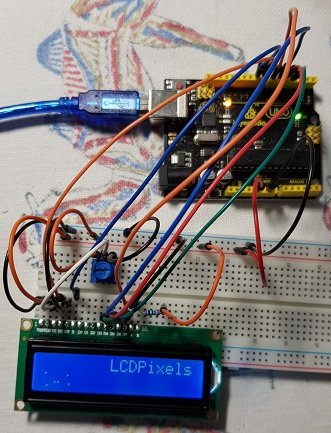Attachments

• b4rLCDBitmapPixels.zip
1.2 KB · Views: 172
•Johan Schoeman

Expert
Toggle between a normal and inverse bitmap...

Sample Code:
B4X:
#Region Project Attributes
#AutoFlushLogs: True
#CheckArrayBounds: True
#StackBufferSize: 300
#End Region

Sub Process_Globals
'These global variables will be declared once when the application starts.
'Public variables can be accessed from all modules.
Public Serial1 As Serial
Dim lcd As LiquidCrystal                                 'rLiquidCrystal must be enabled in the B4R IDE
Dim bitmap As LcdBitmap                                  'rLCDBitmap must be enabled in the B4R IDE
Dim t As Timer

End Sub

Private Sub AppStart
Serial1.Initialize(115200)
Log("AppStart")
t.Initialize("t_tick", 1000)

lcd.Initialize(8,255,9, Array As Byte(4,5,6,7))          'initialize the LCD (rLiquidCrystal) library
lcd.Begin(16,2)
bitmap.Initialize(lcd, 0, 0)
bitmap.begin

lcd.SetCursor(5, 0)
lcd.Write("LCDInverse")

For x = 0 To 3    'This creates a big "X" using the LCDBitmap to show the inverse function
bitmap.line(x, bitmap.BitmapH - 1, x + bitmap.BitmapH - 1, 0, bitmap.COLOR_ON, bitmap.UPDATE_OFF)      'Draw a line, but don't update the bitmap display
bitmap.line(x, 0, x + bitmap.BitmapH - 1, bitmap.BitmapH - 1, bitmap.COLOR_ON, bitmap.UPDATE_OFF)      'Draw a line, but don't update the bitmap display
Next

t.Enabled = True

End Sub

Sub t_tick

bitmap.inverse

End Sub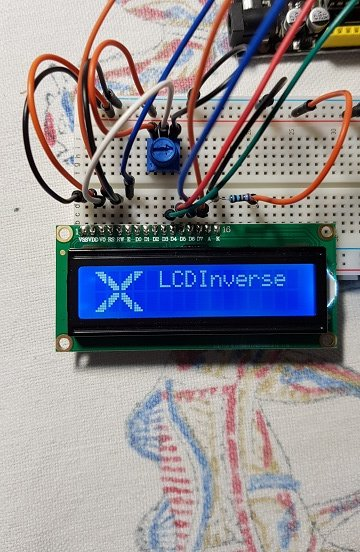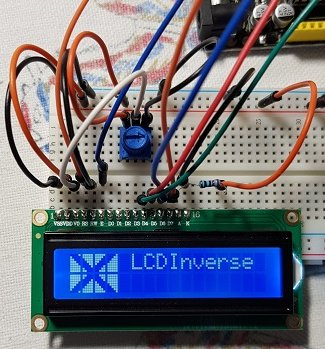Attachments

• b4rLCDBitmapInverse.zip
1.1 KB · Views: 177
•Mostez and Johan Hormaza

B4R Library rLiquidCrystalI2CEx
Replies
0
Views
465
B4R Library rTM1637Ex
Replies
2
Views
568
Replies
3
Views
652
Replies
0
Views
1K
Replies
0
Views
1K